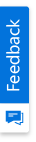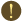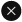We use cookies to give you the best experience on our website. If you continue to browse, then you agree to our privacy policy and cookie policy.Unfortunately, activation email could not send to your email. Please try again.# Set freeze panes in Excel document in C#, VB.NET

Platform: WinForms |
Control: XlsIO
Tags:

Syncfusion Excel (XlsIO) library is a .NET Excel library used to create, read, and edit Excel documents. Also, converts Excel documents to PDF files. Using this library, you can set freeze panes in Excel document.

## How to set freeze panes in Excel?

Rows and columns in an Excel document can be frozen by using the FreezePanes() method of IRange interface. To freeze the rows or columns you need to call FreezePanes() for the range next to the row or column.

For example, if you wish to freeze the first row in Excel sheet, you need to select the range A2. If you select the range B2, then the first row and first column will be frozen in the worksheet. So, you need to select the range next to the range that needs to be frozen.

## Steps to set freeze panes in Excel, programmatically:

Step 1: Create a new C# console application project.Create a new C# console application project

Step 2: Install the Syncfusion.XlsIO.WinForms NuGet package as reference to your .NET Framework application from NuGet.org.Install NuGet package to the project

Step 3: Include the following namespaces in Program.cs file.

C#

```using Syncfusion.XlsIO;
```

VB.NET

```Imports Syncfusion.XlsIO
```

Step 4: Include the following code snippet in main method of Program.cs file to set freeze panes.

C#

```using (ExcelEngine excelEngine = new ExcelEngine())
{
//Instantiate the excel application object
IApplication application = excelEngine.Excel;

//Create a new Excel workbook
IWorkbook workbook = application.Workbooks.Create(1);

//Get the first worksheet of workbook into IWorksheet
IWorksheet worksheet = workbook.Worksheets;

worksheet["A1"].Text = "Id";
worksheet["B1"].Text = "Name";
worksheet["C1"].Text = "Age";

worksheet["A2"].Number = 1;
worksheet["B2"].Text = "Andy Bernard";
worksheet["C2"].Number = 25;

worksheet["A3"].Number = 2;
worksheet["B3"].Text = "Jim Halpert";
worksheet["C3"].Number = 23;

worksheet["A4"].Number = 3;
worksheet["B4"].Text = "Stanley Hudson";
worksheet["C4"].Number = 22;

worksheet.Range["A5"].Number = 4;
worksheet.Range["B5"].Text = "Karen Fillippelli";
worksheet.Range["C5"].Number = 24;

worksheet.Range["A6"].Number = 5;
worksheet.Range["B6"].Text = "Phyllis Lapin";
worksheet.Range["C6"].Number = 27;

//Autofit the coulmns in used range
worksheet.UsedRange.AutofitColumns();

//Apply freeze panes
worksheet.Range["A2"].FreezePanes();

//Save the Excel document
workbook.SaveAs("Output.xlsx");
}
```

VB.NET

```Using excelEngine As ExcelEngine = New ExcelEngine()
'Instantiate the excel application object
Dim application As IApplication = excelEngine.Excel

'Create a new Excel workbook
Dim workbook As IWorkbook = application.Workbooks.Create(1)

'Get the first worksheet of workbook into IWorksheet
Dim worksheet As IWorksheet = workbook.Worksheets(0)

worksheet("A1").Text = "Id"
worksheet("B1").Text = "Name"
worksheet("C1").Text = "Age"

worksheet("A2").Number = 1
worksheet("B2").Text = "Andy Bernard"
worksheet("C2").Number = 25

worksheet("A3").Number = 2
worksheet("B3").Text = "Jim Halpert"
worksheet("C3").Number = 23

worksheet("A4").Number = 3
worksheet("B4").Text = "Stanley Hudson"
worksheet("C4").Number = 22

worksheet.Range("A5").Number = 4
worksheet.Range("B5").Text = "Karen Fillippelli"
worksheet.Range("C5").Number = 24

worksheet.Range("A6").Number = 5
worksheet.Range("B6").Text = "Phyllis Lapin"
worksheet.Range("C6").Number = 27

'Autofit the coulmns in used range
worksheet.UsedRange.AutofitColumns()

'Apply freeze panes
worksheet.Range("A2").FreezePanes()

'Save the Excel document
workbook.SaveAs("Output.xlsx")
End Using
```

A complete working sample of how to set freeze panes in Excel document can be downloaded from Freeze-Panes.zip.

By executing the program, you will get the output Excel file as follows.Output Excel document

Take a moment to peruse the documentation, where you will find other options like move or copy a worksheet, show or hide worksheet and worksheet tabs, page setup settings and more with code examples.

Keep the headers visible while scrolling through data

Click here to explore the rich set of Syncfusion Excel (XlsIO) library features.

Note:

Starting with v16.2.0.x, if you reference Syncfusion assemblies from trial setup or from the NuGet feed, include a license key in your projects. Refer the link to learn about generating and registering Syncfusion license key in your application to use the components without trail message.

2X faster development

The ultimate WinForms UI toolkit to boost your development speed.You are using an outdated version of Internet Explorer that may not display all features of this and other websites. Upgrade to Internet Explorer 8 or newer for a better experience.